Latest Teaching jobs   »   UGC NET Previous Year Question June...

# UGC NET Previous Year Question June 2019 For Logical & Mathematical Reasoning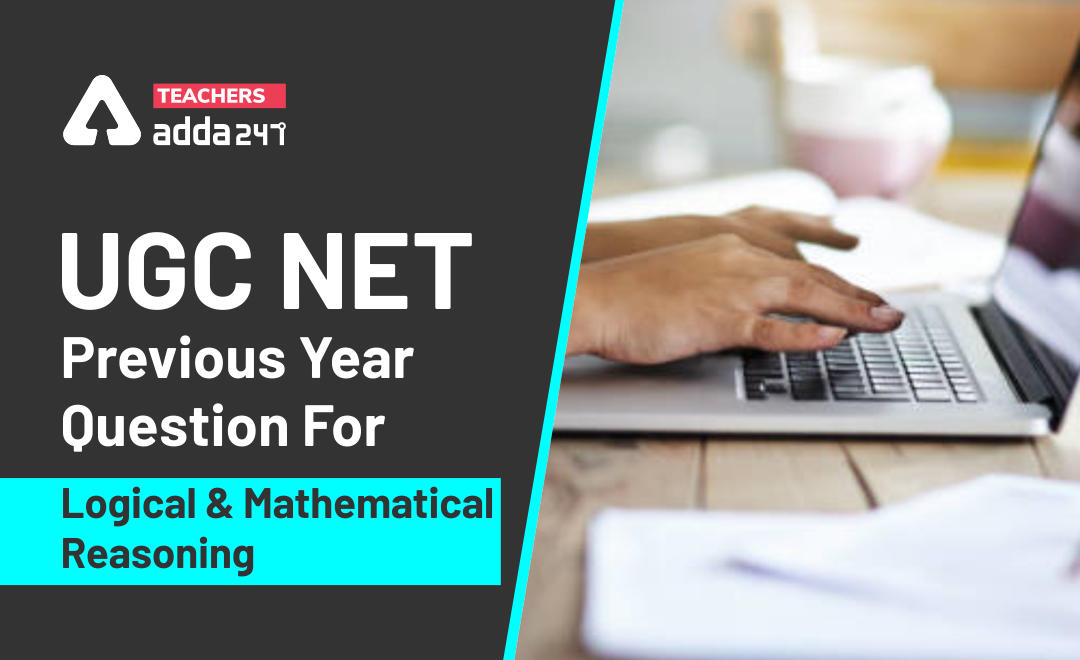Logical & Mathematical Reasoning are  important section in UGC NET Exam 2020.  UGC NET is an entrance exam for the selection of deserving candidates for the posts of Assistant Professor and Junior Research Fellowship (JRF).

Get free study Material for UGC NET 2020

## Highlights :

Exam Name UGC NET
Subject Reasoning (including Maths), Logical Reasoning, Data Interpretation
Total Questions 5 (for each)
Total Marks 10 (for each)
Marking For right answer – 2 mark
Negative Marking No
Question Pattern Objective type
Language Bilingual
Mathematical Reasoning and Aptitude
• Types of reasoning.
• Number series, Letter series, Codes and Relationships.
• Mathematical Aptitude (Fraction, Time & Distance, Ratio, Proportion and Percentage, Profit and Loss, Interest and Discounting, Averages etc.).
##### Logical Reasoning
• Understanding the structure of arguments: argument forms, structure of categorical propositions, Mood and Figure, Formal and Informal fallacies, Uses of language, Connotations and denotations of terms, Classical square of opposition.
• Evaluating and distinguishing deductive and inductive reasoning.
• Venn diagram: Simple and multiple use for establishing validity of arguments.
• Indian Logic: Means of knowledge.
##### Data Interpretation
• Sources, acquisition and classification of Data.
• Quantitative and Qualitative Data.
• Graphical representation (Bar-chart, Histograms, Pie-chart, Table-chart and Line-chart) and mapping of Data.
• Data Interpretation.
• Data and Governance.

Here we are going to provide you quizzes related to UGC NET syllabus which contains mainly flowing topics i.e. Reasoning (including Maths), Logical Reasoning, Data Interpretation

### Today Quiz : UGC NET Previous Yr Quiz June 2019

Q1. Sanjay sold an article at a loss of 25%. If the selling price had been increased by Rs. 175, there would have been a gain of 10%. What was the cost price of the article?
(a) Rs. 350
(b) Rs. 400
(c) Rs. 500
(d) Rs. 750

Q2. A train leaves Agra at 5 am and reaches Delhi at 9 am. Another train leaves Delhi at 7 am and reaches Agra at 10:30 a.m. At what time do the two trains cross each other?
(a) 6:36 am
(b) 6:56 am
(c) 7 am
(d) 7:56 am

Q3. The proposition ‘No red is black is equivalent to which of the following propositions?
(i) No black is red
(ii) All red are black
(iii) Some red are not black
(iv) Red is not black
Select the correct answer from the options given below:
(a) (i), (ii). (iii) and (iv)
(b) (iii) only
(c) (i) and (iv)
(d) (iv) only

Q4. Given below are two premises with four conclusions drawn from them.
Which of the following conclusions could be validly drawn from the premises?
Premises:
(i) Some bags are tables
(ii) All bags are chairs.
Conclusions:
(A) Some tables are chairs
(B) No chair is table
(C) Some chairs are bags
(D) Some bags are not tables
Select the correct answer from the options given below:
(a) (A) and (C)
(b) (B), (C) and (D)
(c) (A) and (D)
(d) (B) only

Q5. The average of 35 raw scores is 18. The average of first seventeen of them is 14 and that of last seventeen is 20. Find the eighteenth raw score.
(a) 42
(b) 46
(c) 52
(d) 56
Q6. Identify the reasoning in the following argument: Pre-active stage of classroom teaching is important just as pre-learning preparation stage of communication’
(a) Hypothetical
(b) Deductive
(c) Inductive
(d) Analogical
Q7. If REASON is coded as 5 and GOVERNMENT as 9, then what is the code for ACCIDENT?
(a) 6
(b) 7
(c) 8
(d) 9

Consider the following table that shows expenditures of a company (in lakh rupees) per annum over the given years. Answer the questions based on the data contained in the table: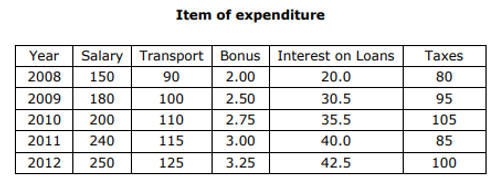Q8. The approximate ratio between the total expenditure on taxes for all the years and the total expenditure on transport for all the years is
(a) 31 : 40
(b) 25: 36
(c) 27 : 30
(d) 31 : 36

Q9. The total amount of bonus paid by the company during the given period is approximately what percent of the total amount of salary paid during this period?
(a) 0.9%
(b) 1.3%
(c) 1.6%
(d) 2.0%
Q10. Total expenditure of all the items in 2008 was approximately what percent of the total expenditure in 2012?
(a) 66%
(b) 69%
(c) 72%
(d) 75%

Q11. The approximate ratio between the total expenditure of the company in the year 2008 and the total expenditure of the company in the year 2009 is
(a) 57: 75
(b) 52: 68
(c) 57 : 68
(d) 68 : 57

Q12. What is the average amount of interest per year which the company had to pay (in Rupees) during this period?
(a) 30.5 Lakh
(b) 32.7 Lakh
(c) 33.7 Lakh
(d) 35.5 Lakh
Solutions
Solutions
S1. Ans.(c)
Sol. Sanjay sold an article at a loss of = 25%.
Acc, to question,
25% + 10% = Rs. 175
100% or Cost Price = 500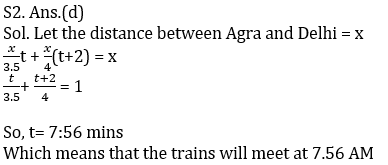S3. Ans.(c)
Sol. (i) No black is red
(iv) Red is not black
S4. Ans.(a)
Sol. (A) Some tables are chairs
(C) Some chairs are bags
S5. Ans.(c)
Sol. Average of 35 raw scores = 18
Total of 35 raw scores = 18 × 35 = 630
total of first seventeen of them = 14×17 = 238
total of last seventeen = 20 × 17 = 340
the eighteenth raw score = 630 – (238 + 340)
= 52
S6. Ans.(d)
Sol. Analogical
S7. Ans.(b)
Sol. no. of alphabets in REASON = 6
REASON is coded as = 6 – 1 = 5
and no. of alphabets in GOVERNMENT = 10
GOVERNMENT is coded as = 10 – 1 = 9
then the code for ACCIDENT = no. of alphabets in ACCIDENT – 1
= 8 – 1 = 7
S8. Ans.(d)
Sol. the total expenditure on taxes for all the years = 80 + 95 + 105 + 85 + 100 = 465
the total expenditure on transport for all the years = 90 + 100 + 110 + 115 + 125 = 540
Required Ratio = 465 : 540
= 31 : 36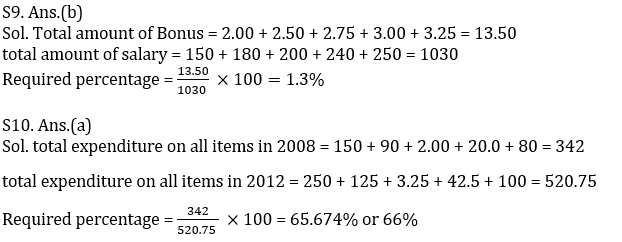S11. Ans.(c)
Sol. total expenditure on all items in 2008 = 150 + 90 + 2.00 + 20.0 + 80 = 342
total expenditure on all items in 2009 = 180 + 100 + 2.50 + 30.5 + 95 = 408
Required ratio = 342 : 408
= 57 : 68
S12. Ans.(c)
Sol. the total amount of interest = 20 + 30.5 + 35.5 + 40.0 + 42.5 = 168.5
the average amount of interest per year = 168.5 ÷ 5 = 33.7

Get free study Material for UGC NET 2020

For More Details :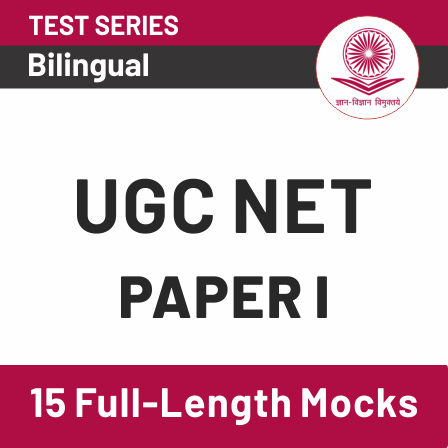Sharing is caring!

Thank You, Your details have been submitted we will get back to you.